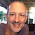### Applied Trigonometry

Of the 29 students in MS 101 Algebra & Trigonometry, 19 were able to correctly calculate 300 sin (45°) and 300 cos(45°). The most common error was making the calculation without specifying degrees. One of the tools used by the class presumes radian inputs by default.

When then asked to calculate the SVG coordinates for the above points, only eight students were successful. All eight had been among the 19 who answered the trigonometry calculation correctly. The result of that calculation is necessary to determining the coordinates above.

The students can make a basic trigonometric calculation but only a few could go on and correctly apply that result to a applied problem in SVG coordinates.

The trigonometric calculation would be a typical student learning outcome in trigonometry, "Students will be able to calculate basic trigonometric values." The SVG coordinates is an actual application of the knowledge, a situation in which the learning is useful and authentic.

1.Applied trigonometry is difficult than trigonometry so your blog will be a blessing for trigonometry lovers, I think math's requires more practice than other subject so one should try to solve math’s problem very well. It will make math interesting and joyful.

1.I have more complete notes on the SVG unit in trigonometry at http://www.comfsm.fm/~dleeling/math/mb2/notes.html#page-62